Electromagnetic Waves   Share

What is Electromagnetic Waves

Wave is nothing but a pattern of disturbance which propagates and carry energy with it. You can produce a wave on a rope by moving one end of the rope up and down. The wave produces on rope needs a medium to propagate and here medium is rope itself. This type of waves is known as mechanical waves. But in the case of Electromagnetic waves, they don't need a medium to propagate. Electromagnetic waves are waves that are created as a result of variations of electric field and a magnetic field. Or we can say that Electromagnetic waves are nothing but changing magnetic and electric fields. Electromagnetic waves are also known to be solutions of Maxwell's equations. And Maxwell's equations are the fundamental equations of electrodynamics. Electromagnetic waves can transmit energy and travel through a vacuum. light waves are examples of electromagnetic waves. Generally, Electromagnetic waves are shown by a sinusoidal graph.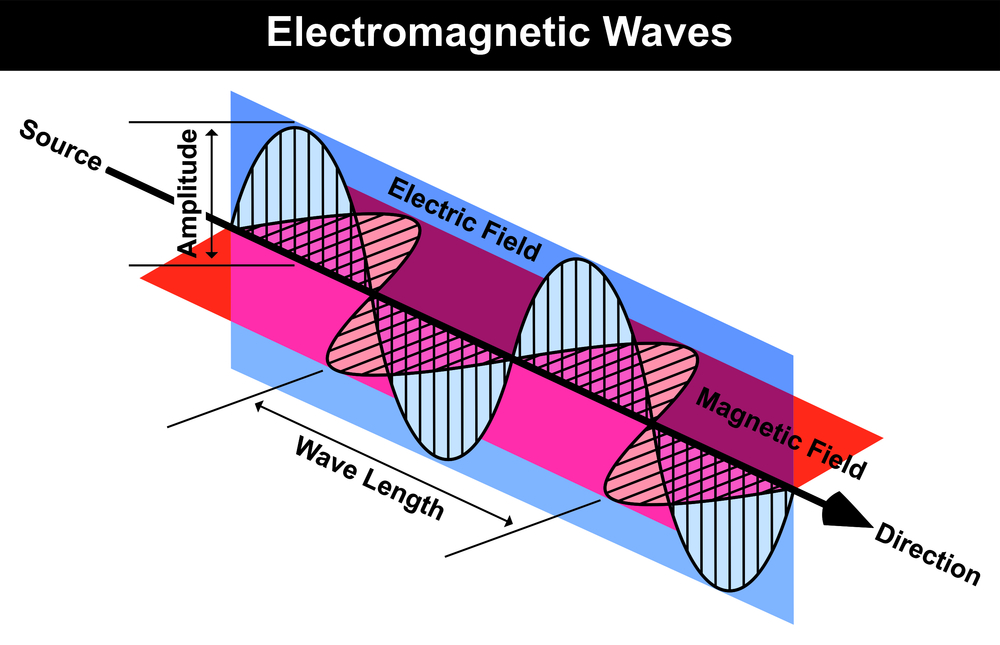As shown in figure Electromagnetic waves consist of time-varying electric and magnetic fields and they are perpendicular to each other And these both fields are also perpendicular to the direction of propagation of waves. And because of this Electromagnetic waves are transverse in nature.

Electromagnetic waves are one of the most important chapters from modern physics while preparing for all competitive exam because it helps you to understand the important properties of Electromagnetic waves. This chapter will also help you to understand various types of Electromagnetic waves and their applications. This is easy to understand and a high scoring topic. Sometimes the Concept of Electromagnetic waves and other chapters of physics are mixed in miscellaneous questions which are asked in various competitive exams.

Prepare Online for JEE Main/NEET

Crack JEE 2021 with JEE/NEET Online Preparation Program

Notes on the Electromagnetic waves

So we will discuss step by step about important topics from this chapter followed by an overview of this chapter. Then we will understand important formulas from this chapter. Remembering these formulas will increase your speed while question-solving.

Electromagnetic waves Topics

• Electromagnetic waves and their characteristics. Transverse nature of electromagnetic waves.

• Electromagnetic spectrum (microwaves, infrared, visible, radio waves, X-rays, ultraviolet, gamma rays). Applications of e.m. waves.

Electromagnetic waves Overview

In Electromagnetic waves chapter, we will learn Electromagnetic waves, their all the important properties, their types, and their applications. So we will learn about

• Maxwell’s Equations- As Electromagnetic waves are also solutions of Maxwell's equations. So it important to learn about them. James C. Maxwell noticed an inconsistency in Ampere’s Law and an asymmetry in the laws of electromagnetism. So he provided some suggestions in these laws. For example, he suggested the idea of Displacement current to remove this inconsistency in Ampere’s Law. Thus he provided a modified version of these laws with the help of Maxwell's equations which are given below-

1. Maxwell's 1st equation -Gauss's Law for electrostatics

2. Maxwell's 2nd equation: Gauss’s Law in Magnetism

4. Maxwell's 4th equation-Ampere's law

• Electromagnetic waves properties-

1. These waves do not require a medium for propagation and they are transverse in nature.

2. These waves propagate through space with the speed of light in vacuum.

3. The wave having higher the frequency,  will have the higher energy associated with it.

4. It can be used to carry information.

5. It travels in straight lines.

6. It can be split and recombined to form interference / Diffraction pattern.

7. It can be reflected or refracted.

• Electromagnetic Wave Equation-

One of the very important types of electromagnetic waves is sinusoidal plane waves. All electromagnetic waves can be considered as a linear superposition of sinusoidal plane waves traveling in arbitrary directions.

For example- A plane wave traveling in the x-direction is of the form

And if E is in the y-z plane then

Where  E - Electric field at (x,t)

- Electric field amplitude

= Angular frequency

c= Speed of light in vacuum

Similarly, B is in the y-z plane

Where B = Magnetic field at (x,t)

= Magnetic field amplitude

• The important physical quantity of Electromagnetic waves

You will learn about some physical quantity given below-

1. The intensity of Electromagnetic wave

2. The momentum of Electromagnetic wave

3. The energy density of Electromagnetic wave

4. The wavelength of Electromagnetic Wave

5. Frequency of Electromagnetic wave

Types of Electromagnetic wave and their applications

Depending on the range of frequency of wave there are various types of Electromagnetic wave. And these waves have various applications in our day to day life. Following are some types of Electromagnetic wave

1. γ - Ray (Gamma Ray)- Useful in In detecting a fault, crack, flaws, holes in metals.

2. X Rays- Useful in X-Ray therapy.

3. Ultraviolet Radiation- Helps In the study of molecular structure.

4. Infrared Waves- Helps for taking photographs in fog or smoke

5. Microwaves-These are used in radio and TV communication.

Electromagnetic Waves Formulas

• Wave Equation-

E is in the y-z plane

B is in the y-z plane

• Maxwell's Displacement Current-

• Gauss's Law for electricity-

• Gauss's law for magnetism-

• Ampere's law-

• The relation between     and  -

• The energy density of EM wave-

• The intensity of EM wave-

• The momentum of EM wave-

How to prepare Electromagnetic waves

• First, you should have good command over the concepts of Electromagnetic waves and you should also know how to apply them well at the time of the entrance exam or while solving questions.
• Please try to understand each concept from this chapter, with the help of theory, questions with solutions and video lectures on each important concept.
• For each concept practice enough problems so that you have a thorough understanding of the concept.
• Solve all the questions at home with proper concentration and try to do all calculation by yourself without seeing the solution first.
• While solving questions of wave equation you should also consider the direction of corresponding vectors.
• Please have a thorough knowledge of Electromagnetic wave spectrum and application of various Electromagnetic waves.
• Remember all the formulas of Energy, momentum, frequency of Electromagnetic waves. This will help you while solving questions.

Electromagnetic Waves Tips

• Make a plan to prepare for this chapter and Stick to a Timetable.
• Make smart and realistic goals and try to achieve them.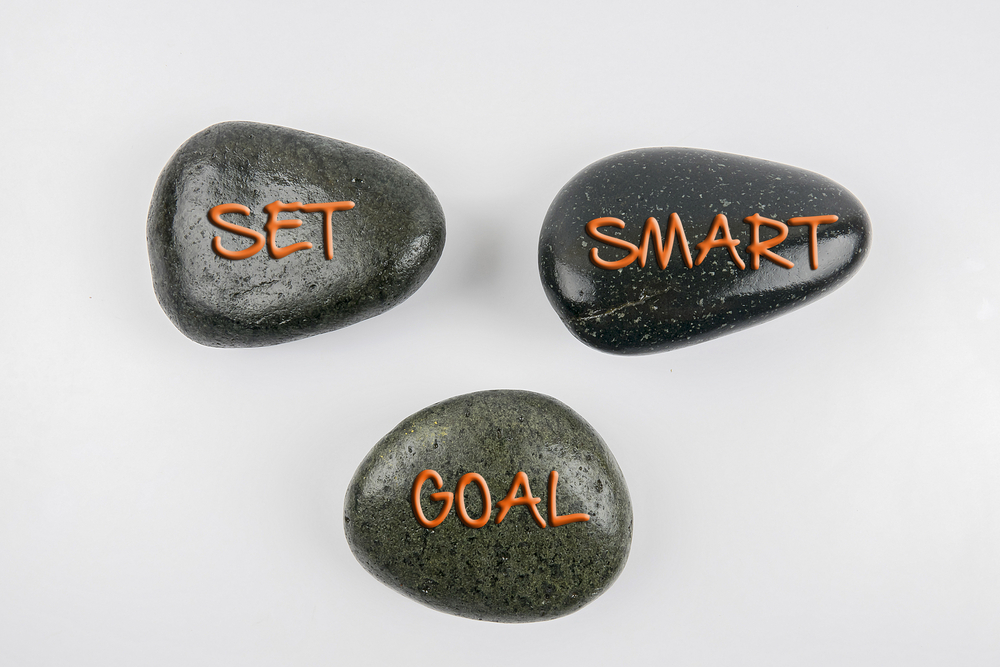• Study the concept first and then start solving questions. Don't go through question directly without knowing the concept.
• Formulas from this chapter are very important from the point of view of competitive exam. So please remember them and solve lots of questions based on these formulas.
• Solve previous year question of various exam from this chapter.
• Use smart methods to solve questions.

Electromagnetic Waves Books

For  Electromagnetic Waves, chapter concepts in NCERT are enough but you will have to practice lots of questions including previous year questions and you can follow other standard books available for competitive exam preparation like Concepts of Physics (H. C. Verma) and Understanding Physics by D. C. Pandey (Arihant Publications).

Physics Chapter wise Notes For Engineering and Medical Exams

 Chapters No. Chapters Name Chapter 1 Physics and Measurement Chapter 2 Kinematics Chapter 3 Laws of motion Chapter 4 Work Energy and Power Chapter 5 Rotational Motion Chapter 6 Gravitation Chapter 7 Properties of Solids and Liquids Chapter 8 Kinetic theory of Gases Chapter 9 Thermodynamics Chapter 10 Oscillations and Waves Chapter 11 Electrostatics Chapter 12 Current Electricity Chapter 13 Magnetic Effects of Current and Magnetism Chapter 14 Electromagnetic Induction and Alternating currents Chapter 16 Optics Chapter 17 Dual Nature of Matter and Radiation Chapter  18 Atoms And Nuclei Chapter 19 Electronic devices Chapter 20 Communication Systems Chapter 21 Experimental skills

Topics from Electromagnetic Waves

• Electromagnetic waves and their characteristics ( JEE Main ) (27 concepts)
• Transverse nature of electromagnetic waves. ( JEE Main ) (10 concepts)
• Electromagnetic spectrum (radio waves, microwaves, infrared, visible, ultraviolet, Xrays, gamma rays). ( JEE Main ) (13 concepts)
• Maxwell's Displacement Current ( JEE Main ) (2 concepts)
• Electromagnetic Waves and their properties ( JEE Main ) (3 concepts)

Important Books for Electromagnetic Waves

•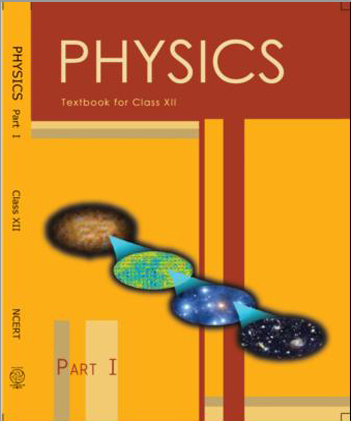•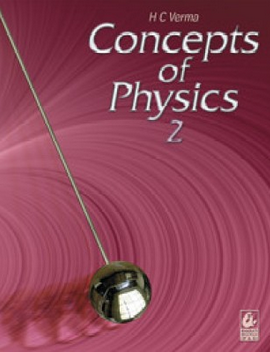•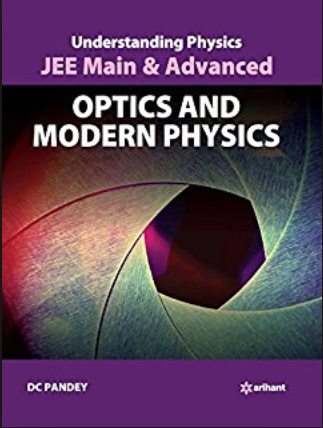Exams
Articles
Questions